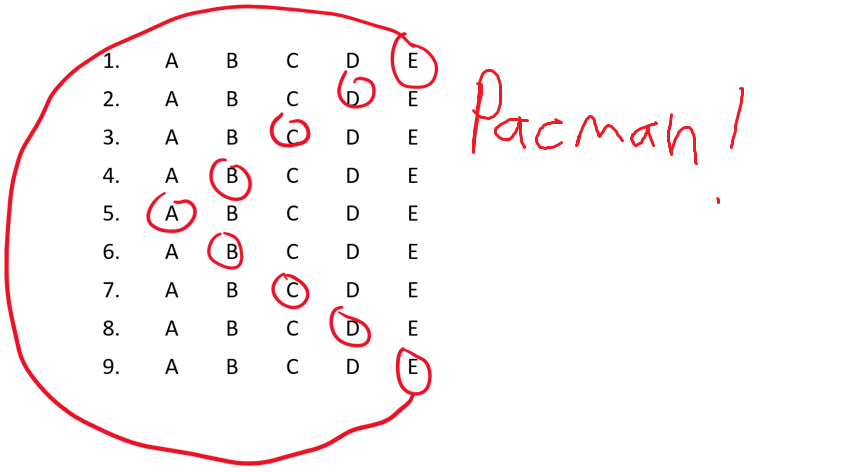# Want to know if your students are taking conceptual inventories seriously? There’s a test for that.

Authors: David Waters, Dragos Amarie, Rebecca A. Booth, Christopher Conover, and Eleanor C. Sayre

First author’s institution: St Louis College of Pharmacy

Journal: Physical Review Physics Education Research 15, 020118 (2019)

Conceptual inventories are a key component of assessing learning in physics. For example, many studies use conceptual inventories to compare the amount students learned under one curriculum to another. Conceptual inventories after all are one of the ways we know active learning results in better learning than regular lectures. Yet, all of this research assumes that students are actually giving an honest effort on the conceptual inventories. Often, conceptual inventories are given in recitations for participation credit and hence, there is no incentive for the student to try to answer the questions correctly. Prior work has found that if a conceptual inventory is not graded for correctness, around 3% of the students may not be taking the test seriously. The researchers came up with that number through longitudinal studies and counting the number of students who left most of the responses blank or gave the same response to most items. But what if you wanted to estimate how many students are putting in an honest effort on a conceptual inventory for your own class? Today’s paper tries to answer that question.

The authors of today’s paper laid out three possible tests. First, they added additional criteria to a technique used in a previous study, which they referred to as the pattern recognition test. If a student were not taking a test seriously, they may leave most of the questions blank, provide the same answer multiple times, or create some pattern in their responses. For example, the authors considered eight or more instances of the same letter response to be indicative of not taking the conceptual inventory seriously. For the patterns, the researchers considered 3 instances of ABCD, 2 instances of ABCDE, or 1 instance of ABCDEDCBA to be indicative of the student not taking the test seriously. For all of the conceptual inventories the authors would later apply their tests to, none of the these patterns would appear if the student answered most questions correctly.

Second, the authors defined a test called the uncommon answer test. Conceptual inventories are designed to test how much students have learned and hence, contain distractor answers that sound reasonable. For example, suppose there is a question in which a ball is thrown straight up in the air and then comes down and the student is asked to find the acceleration when the ball is at its highest point. Since the ball is momentarily at rest at its highest point, the student may think the acceleration is zero since the ball isn’t moving. Therefore, a common incorrect answer would be the acceleration is zero instead of 9.8 m/s2 (assuming we are on Earth). However, not all of the possible answer choices will be picked by a lot of students. If a student were to pick multiple of these uncommon answers, it is possible that the student is not trying their best. To make this test quantitative, the authors decided that if a student picked at least 3 or 4 uncommon answers out of a set of 9 questions where most of the other students picked between only 2 or 3 of the possible responses, that student may not be taking the conceptual inventory seriously.

Finally, the authors defined a test called the easy question test. For each conceptual inventory the authors looked at, they selected the questions the students answered correctly most often. By plotting the results (fig 1), the authors found that the number of students who get all the easy questions incorrect doesn’t change after looking at four questions. Therefore, the authors only considered the four questions the students performed best on. Since these are the questions that were answered correctly most often, any student who was trying should get at least one of them correct. Therefore, the authors decided if a student answered none of these correctly, the student may not be taking the test seriously.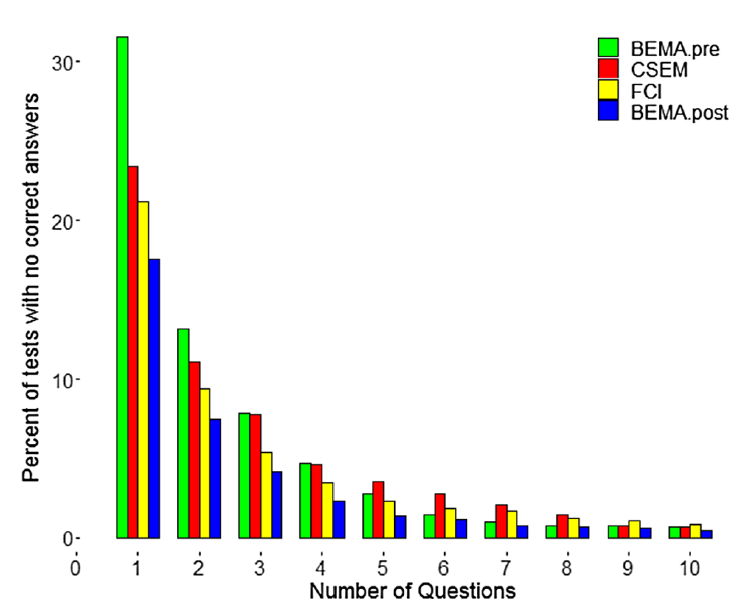Figure 1: The percent of students with no correct answers based on the number of questions looked at. Above four questions, the percent of student is about the same. (Fig 1 in paper).

To actually test their tests, the authors used data collected from PhysPort, which is an online platform for collecting and scoring physics conceptual inventories. For this study, the authors focused on three common conceptual inventories: the Force Concept Inventory (FCI), the Conceptual Survey of Electricity and Magnetism (CSEM), and the Brief Electricity and Magnetism Assessment (BEMA). Across all three conceptual inventories, the authors had access to answers from over 85,000 students.

To see how well their tests could identify students who may not be taking the conceptual inventories seriously, the authors simulated responses from 20,000 students as if they were randomly guessing on each question. If the tests worked as intended, all of these simulated students should have been found to be not taking the conceptual inventories seriously.

So what did the authors find? Overall, the authors found that the percentage of students not taking the conceptual inventories was small, likely only a few percent. For example, the easy questions test found that 4.6% (3.6+0.7+.0.2+0.1) of students taking the CSEM may not have taken it seriously (figure 2).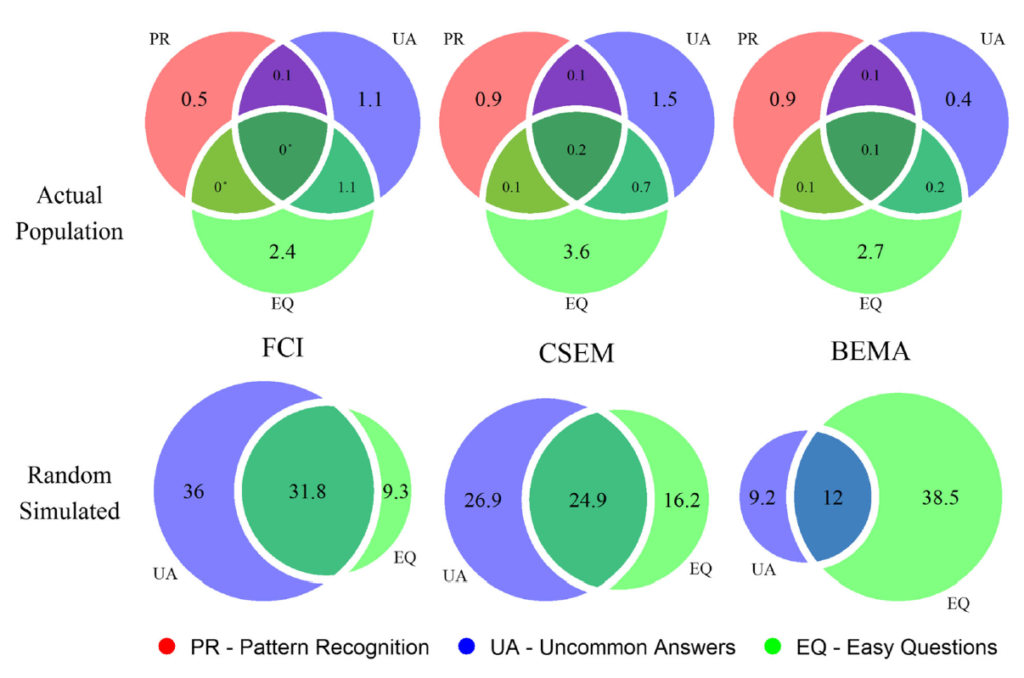Figure 2: Percentage of students identified as not giving an honest effort by each test. (Fig 3 in paper)

In contrast, the easy questions test identified at least 40% of the simulated students as not taking the conceptual inventories seriously. However, there was not a large overlap among the three tests, as the largest percentage of students identified by all three tests was 0.2% on the CSEM. Since there was the less amount of overlap between the pattern recognition test and the other two, the authors decided that a non-serious student is one who fails either the pattern recognition test or the uncommon answer test and the easy question test. Under this decision, the overall estimated percentage of students who did not take the conceptual inventory seriously is between 1.5% and 2.2%.

As the uncommon answer test and the easy question test were the only tests to identify the simulated students as not trying, the authors wanted to see how the threshold for the tests may change the results. For example, the authors had originally picked 3 or 4 uncommon answers as the threshold for not giving a serious effort. When looking at figure 3, it appears that this was a reasonable choice. For each of the conceptual inventories, most of the simulated students would fail the uncommon answer test at this threshold while very few of the actual students do.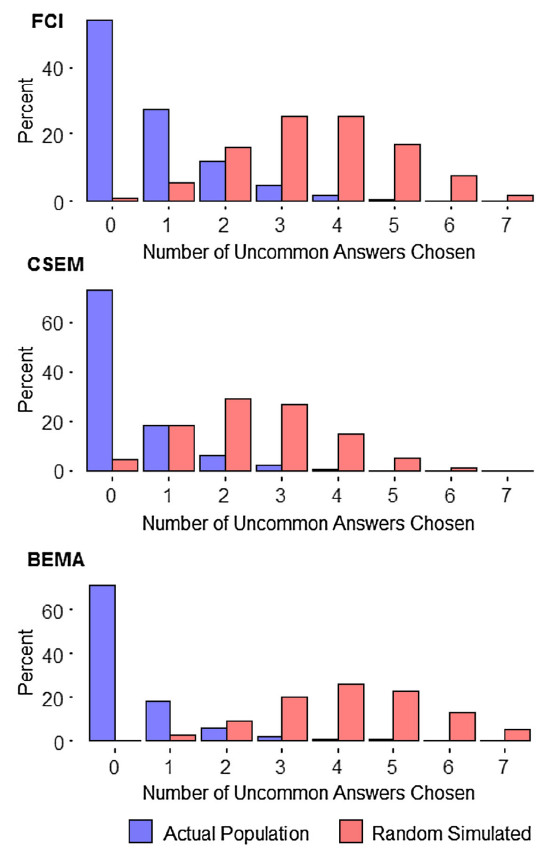Figure 3: The percent of students choosing a certain number of uncommon answers. Notice the differences in distributions between the actual students and the simulated students. (Fig 4 in paper).

On the other hand, the threshold for the easy questions was likely too low as around 40% of the simulated students would not have failed the easy question test simply by randomly guessing an answer (figure 4). As the authors note, this results in an underestimate of the number of students who may not be taking the conceptual inventories seriously.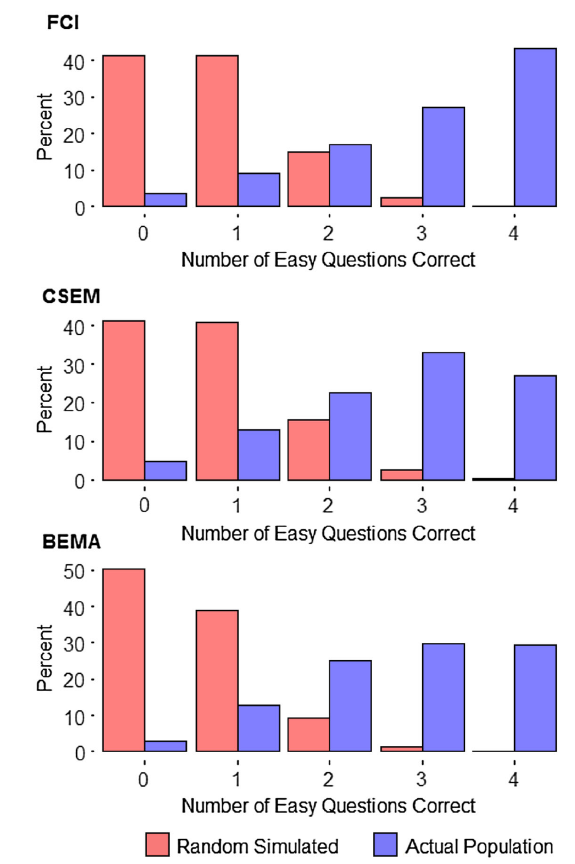Figure 4: The percent of students answering the “easy’ questions correctly. Again, notice the difference in distributions between the actual students and the simulated students. (Fig 5 in paper).

Overall, the three tests developed suggest that even if conceptual inventories are only given for participation credit, only a few percent of the students will not give an honest effort. That is, not grading conceptual inventories for accuracy does not seem to limit their validity. Despite the tests focusing on individual students, the authors caution using their tests to identify students who may not have given an honest effort. Instead, researchers and instructors should use the tests at the course level, focusing on the fraction of students who may not have given an honest effort.